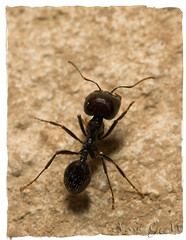# Langton’s Ant in Python using PygletSome months ago I have been reading a bit about Turmites. This term is used to describe Turing machines that use a two-dimensional grid of cells as a tape. The term was coined because these Turing machines operating on the grid look like insects.

There’s an interesting turmite named “Langton’s Ant“, which is also a Cellular Automata. It consists of a two-dimensional grid of cells;  each cell has two possible states: black or white. There’s also an ant involved, which moves along the grid. When the ant leaves a cell, it inverts the color of the cell. In this post we’ll see how to implement the Langton’s Ant in Python using Pyglet.

The rules that govern the behavior of the Langton’s Ant are very simple:

• At a white cell, turn 90° right, flip the color of the cell, move forward one unit
• At a black cell, turn 90° left, flip the color of the cell, move forward one unit

It will be easier to understand with some pictures. This is the look of the grid after 424 steps. The red square is the ant.

This is how it looks after 2806 steps:

And after 4607 iterations:

A characteristic of the Langton’s Ant is that, when starting with an empty grid, and after performing around 10000 steps, the ant will eventually build a pattern called a “highway”, which looks like stairs to me.

This is how the grid looks after  ~10700 steps. Note the “highway” pattern in the lower part of the image:

There’s a Python implementation of the Langton’s Ant available on the Internet that uses the well-known Pygame library, and I decided to port it to Pyglet. Pyglet is a cross-platform multimedia library for Python.

The implementation code follows. Unfortunately, the free WordPress hosting does not support plugins, so there’s no syntax highlighting here 😦

EDIT: Thanks to Pastafr0la for helping me to post source code with syntax highlighting!

```# -*- coding: utf-8 -*-

# Rules (http://en.wikipedia.org/wiki/Langton%27s_ant)
#
# At a white square, turn 90º right, flip the color of the square, move forward one unit
# At a black square, turn 90º left, flip the color of the square, move forward one unit
#

import pyglet
from pyglet import window
from pyglet import clock
from pyglet import font

#Dimensions of the screen
window_width  = 800
window_height = 600
cell_size = 10
columns = window_width / cell_size
rows = window_height / cell_size

print "columns: %d" % columns
print "rows: %d" % rows

class Ant:
def __init__(self, posx, posy):
#Initial position of the ant
self.posx = posx
self.posy = posy
self.dir = 2
self.dirs = ((-1, 0),
(0, 1),
(1, 0),
(0, -1)
)

def turn(self, direction):
if direction == 'right':
self.dir = (self.dir + 1) % 4
elif direction == 'left':
self.dir = (self.dir + 3) % 4

self.posx = (self.posx + self.dirs[self.dir]) % columns
self.posy = (self.posy + self.dirs[self.dir]) % rows

class Grid(pyglet.window.Window):

def __init__(self, width, height):
#Let all of the standard stuff pass through
window.Window.__init__(self, width=width, height=height)

#Initial position of the ant: middle of the grid
self.ant = Ant(columns/2, rows/2)

#False = black cell. True = white cell.
self.cells = [[False] * columns for i in range(rows)]
self.steps = 0

def rectangle(self, x1, y1, x2, y2):
pyglet.graphics.draw(4, pyglet.gl.GL_QUADS, ('v2f', (x1, y1, x1, y2, x2, y2, x2, y1)))

def draw_grid(self):
pyglet.gl.glColor4f(0.23, 0.23, 0.23, 1.0)
#Horizontal lines
for i in range(rows):
pyglet.graphics.draw(2, pyglet.gl.GL_LINES, ('v2i', (0, i * cell_size, window_width, i * cell_size)))
#Vertical lines
for j in range(columns):
pyglet.graphics.draw(2, pyglet.gl.GL_LINES, ('v2i', (j * cell_size, 0, j * cell_size, window_height)))

def draw(self):
self.clear()

#White color for white cells
pyglet.gl.glColor4f(1.0, 1.0, 1.0, 1.0)

for f in range(len(self.cells)):
current_row = self.cells[f]
for c in range(len(current_row)):
if current_row[c]:
self.rectangle(c * cell_size, f * cell_size,
c * cell_size + cell_size, f * cell_size + cell_size)

self.draw_grid()

#ant's color
pyglet.gl.glColor4f(1.0, 0.23, 0.23, 1.0)

self.rectangle(self.ant.posx * cell_size, self.ant.posy * cell_size,
self.ant.posx * cell_size + cell_size, self.ant.posy * cell_size + cell_size)

def main_loop(self):
#Create a font for our Steps label
#The pyglet.font.Text object to display the steps
steps_text = font.Text(ft, y=10, color=(1.0, 0.0, 0.0, 1.0))

clock.set_fps_limit(60)

while not self.has_exit:
self.dispatch_events()

self.move()
self.draw()

#Tick the clock
clock.tick()
#Show the number of steps performed by the ant
steps_text.text = "Steps: %d" % self.steps
steps_text.draw()
self.flip()

def move(self):
#Is the ant on a white cell? =&gt; Make the cell black, turn 90º right, move forward one cell
if self.cells[self.ant.posy][self.ant.posx]:
self.cells[self.ant.posy][self.ant.posx] = False
self.ant.turn('right')
#Is the ant on a black cell? =&gt; Make the cell white, turn 90º left, move forward one cell
else:
self.cells[self.ant.posy][self.ant.posx] = True
self.ant.turn('left')

self.steps += 1

if __name__ == "__main__":
h = Grid(window_width, window_height)
h.main_loop()```
1.NCR says:
2.jay bolton says: